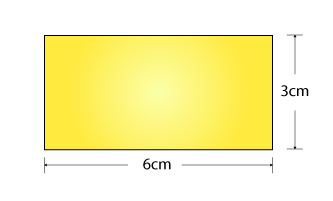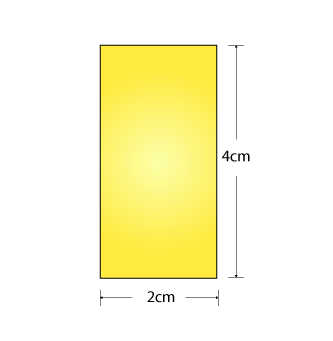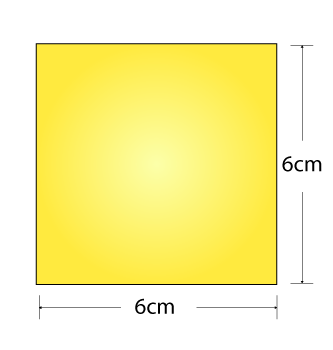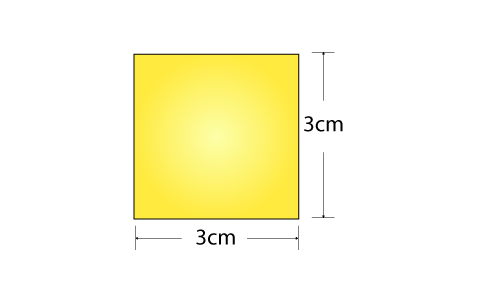# Scale Factor

Scale Factor is used to scale shapes in different dimensions. In geometry, we learn about different geometrical shapes which both in two-dimension and three-dimension. The scale factor is a measure for similar figures, who look the same but have different scales or measures. Suppose, two circle looks similar but they could have varying radii.

The scale factor states the scale by which a figure is bigger or smaller than the original figure.  It is possible to draw the enlarged shape or reduced shape of any original shape with the help of scale factor.

## What is the Scale factor

The size by which the shape is enlarged or reduced is called as its scale factor. It is used when we need to increase the size of a 2D shape, such as circle, triangle, square, rectangle, etc.

If y = Kx is an equation, then K is the scale factor for x. We can represent this expression in terms of proportionality also:

∝ x

Hence, we can consider K as a constant of proportionality here.

The scale factor can also be better understood by Basic Proportionality Theorem.

## Scale Factor Formula

The formula for scale factor is given by:

Dimensions of Original Shape x scale Factor = Dimension of new shape

Scale factor = Dimension of New Shape/Dimension of Original Shape

Take an example of two squares having length-sides 6 unit and 3 unit respectively. Now, to find the scale factor follow the steps below.

Step 1: 6 x scale factor = 3

Step 2: Scale factor = 3/6 (Divide each side by 6).

Step 3: Scale factor = ½ =1:2(Simplified).

Hence, the scale factor from the larger Square to the smaller square is 1:2.

The scale factor can be used with various different shapes too.

## Scale Factor Problem

For example, there’s a rectangle with measurements 6 cm and 3 cm.Both sides of the rectangle will be doubled if we increase the scale factor for the original rectangle by 2. I.e By increasing the scale factor we mean to multiply the existing measurement of the rectangle by the given scale factor. Here, we have multiplied the original measurement of the rectangle by 2.

Originally, the rectangle’s length was 6 cm and Breadth was 3 cm.

After increasing its scale factor by 2, the length is 12 cm and Breadth is 6 cm.

Both sides will be triple if we increase the scale factor for the original rectangle by 3. I.e By increasing the scale factor we mean to multiply the existing measurement of the rectangle by the given scale factor. Here, we have multiplied the original measurement of the rectangle by 3.

Originally, the rectangle’s length was 6 cm and Breadth was 3 cm.

After increasing its scale factor by 3, the length is 18 cm and Breadth is 9 cm.

## How to find the scale factor of Enlargement

Problem 1: Increase the scale factor of the given Rectangle by 4.Hint: Multiply the given measurements by 4.

Solution: Given Length of original rectangle = 4cm

Width or breadth of given rectangle = 2cm

Now as per the given question, we need to increase the size of the given triangle by scale factor of 4.

Thus, we need to multiply the dimensions of given rectangle by 4.

Therefore, the dimensions of new rectangle or enlarged rectangle is given by:

Length = 4 x 4 = 16cm

And Breadth = 2 x 4 = 8cm.

### Scale Factor of 2

The scale factor of 2 means the new shape obtained after scaling the original shape is twice of the shape of the original shape.

The example below will help you to understand the concept of scale factor of 2.

Problem 2: Look at square Q. What scale factor has square P increased by?Hint: Work Backwards, and divide the measurements of the new triangle by the original one to get the scale factor.

Solution: Divide the length of one side of the larger square by the scale factor.

We will get the length of the corresponding side of the smaller square.

## Scale Factor of Triangle

The triangles which are similar have same shape and measure of three angles are also same. The only thing which varies is their sides.  However, the ratio of the sides of one triangle is equivalent to the ratio of sides of another triangle, which is called here the scale factor.

If we have to find the enlarged triangle similar to the smaller triangle, we need to multiply the side-lengths of the smaller triangle by the scale factor.

Similarly, if we have to draw a smaller triangle similar to bigger one, we need to divide the side-lengths of the original triangle by scale factor.

## Real-life Applications of Scale Factor

It is important to study real-life applications to understand the concept more clearly:

Because of various numbers getting multiplied or divided by a particular number to increase or decrease the given figure, scale factor can be compared to Ratios and Proportions.

1. If there’s a larger group of people than expected at a party at your home. You need to increase the ingredients of the food items by multiplying each one by the same number to feed them all. Example, If there are 4 people extra than you expected and one person needs 2 pizza slices, then you need to make 8 more pizza slices to feed them all.
2. Similarly, the Scale factor is used to find a particular percentage increase or to calculate the percentage of an amount.
3. It also lets us work out the ratio and proportion of various groups, using the times’ table knowledge.
4. To transform Size: In this, the ratio of expressing how much to be magnified can be worked out.
5. Scale Drawing: It is the ratio of measuring the drawing compared to the original figure given.
6. To compare 2 Similar geometric figures: When we compare two similar geometric figures by the scale factor, it gives the ratio of the lengths of the corresponding sides.